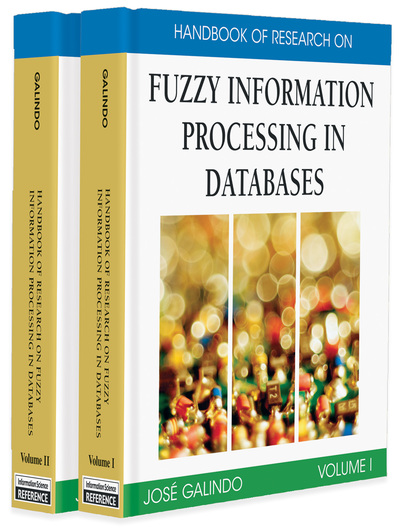# Evaluation of Quantified Statements Using Gradual Numbers

Ludovic Liétard, Daniel Rocacher
DOI: 10.4018/978-1-59904-853-6.ch010
OnDemand:
(Individual Chapters)
Available
\$29.50
No Current Special Offers

## Abstract

This chapter is devoted to the evaluation of quantified statements which can be found in many applications as decision making, expert systems, or flexible querying of relational databases using fuzzy set theory. Its contribution is to introduce the main techniques to evaluate such statements and to propose a new theoretical background for the evaluation of quantified statements of type “Q X are A” and “Q B X are A.” In this context, quantified statements are interpreted using an arithmetic on gradual numbers from Nf, Zf, and Qf. It is shown that the context of fuzzy numbers provides a framework to unify previous approaches and can be the base for the definition of new approaches.

## Key Terms in this Chapter

Fuzzy Predicate: Predicate defined by a fuzzy set. A fuzzy predicate delivers a degree of satisfaction.

Linguistic Quantifiers: Quantifiers defined by linguistic expressions like “around 5” or “most of.” Such quantifiers allow an intermediate attitude between the conjunction (expressed by the universal quantifier ?) and the disjunction (expressed by the existential quantifier ?).

Gradual Integer: Integer which takes the form of a fuzzy subset of the set of naturals (interpreted as a conjunction). Such integers differ from fuzzy numbers which are interpreted as disjunctions of candidates.

Gradual Relative Integer: Gradual number represented by a fuzzy subset of the set of relatives (interpreted as a conjunction). It is defined as the substraction of two gradual integers.

Sugeno Fuzzy Integral: Aggregate operator which can be viewed as a compromise between two aspects: (1) a certain quantity (a fuzzy measure) and (2) a quality of information (a fuzzy set).

OWA Operator: Ordered Weighted Average Operator. The inputs are assumed to be sorted and the weights of this average are associated to input data depending on their rank (weight w1 is associated to the largest input, weight w2 is associated to the second largest input, and so forth).

Gradual Relational Number: Gradual number interpreted as a conjunction and defined as the ratio of two relative integers.

## Complete Chapter List

Search this Book:
Reset